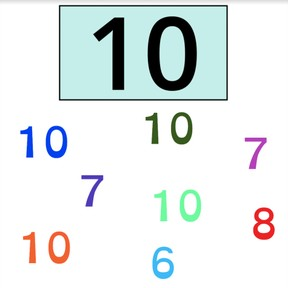Recognizing numbers to 10

# Recognizing numbers to 10

Recognizing numbers to 10

No account needed.8,000 schools use Gynzy92,000 teachers use Gynzy1,600,000 students use Gynzy

## General

Students recognize the numbers from 0-10 when presented in numerical form.

K.CC.A.3

## Relevance

Discuss with students that you come across numbers all over the place and it is important to be able to recognize them. An example would be using numbers when they learn to tell time, or to know what the date is.

## Introduction

Ask students to discuss where they see numbers in pairs and have them share a few examples with the class.

## Development

The student points to the numbers from 0-10 and says their names aloud. Next the numbers appear in random order. Point at a number and have students find and hold up the matching number card. Check that the students recognize 6,7, and 8 by circling the correct numbers.

Check that students understand by asking the following questions:
Where might you see these numbers?
You can choose to write a few numbers on the interactive whiteboard and ask students to find the matching number card.

## Guided Practice

Students practice recognizing numbers. First they select all numbers with a 5, next they look for pairs of numbers and dragging them to the right box and finally by typing the number given.

## Closing

Discuss with students the importance of recognizing numbers to 10. Numbers are everywhere. It can be useful when learning to read a clock, or to tell the date. Check that students have understood by dragging the blue numbers to the right place. Students search for a number pair.Finally the students are asked to circle the numbers in the image, without explaining the function or meaning of the number.

## Teaching Tip

Students with strong numbers skills can be asked to name the numbers to 10, for a challenge they can work with numbers to 20.

## Instruction materials

Number cards from 0-10.

### The online teaching platform for interactive whiteboards and displays in schools

• Save time building lessons

• Manage the classroom more efficiently

• Increase student engagement